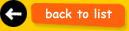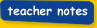Thursday 20th June 2019You are in:Classroom Keystage 1·Maths·Calculations·Addition and SubtractionAddition and Subtraction Facts up to 10 - 1Subtraction reverses addition sums (Subtraction is the inverse of addition) - look at this example: 8 + 1 = 9       9 - 1 = 8Type in the correct answer to these addition sums.0 + 9 =           5 + 4 = 1 + 8 =           6 + 3 = 2 + 7 =           7 + 2 = 3 + 6 =           8 + 1 = 4 + 5 =           9 + 0 = What do you notice about the pattern of the answers?  Answers go down by one All answers are the same Answers go up in one